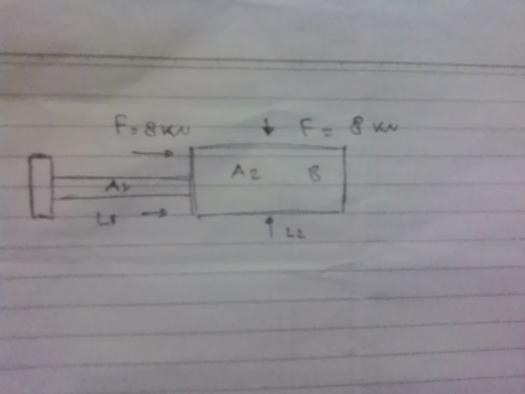# Beam Deflectionhdr.jpg" />

this beam made of steel with moduus youg of 300 gpa, cross sectional area A1 =10.10^-6 m2
A2 = 20. 10^-6 m2.
length L1 = 2m
Length L2 =1,8 m.

at he level that is very rigid ring mounted to apply the load F=8 KN.

questions :
1.calculate reaction pedestal points A and B
2. stresses that ocur in cross section A1 and A2
3. the displacement Point C.

please help me, I've spent two weeks to do .. I say many thanks to friends in this forum

• Mathematics inside the configured delimiters is rendered by MathJax. The default math delimiters are $$...$$ and $...$ for displayed mathematics, and $...$ and $...$ for in-line mathematics.